# RD Sharma Solutions For Class 7 Maths Chapter 17 Constructions Exercise 17.1

Students can refer to RD Sharma Solutions for Class 7 Maths Exercise 17.1 Chapter 17 Constructions which are available here. The solutions here are solved step by step for a better understanding of the concepts, which helps students prepare for their exams with ease. Our expert tutors at BYJU’S have made it possible to help students crack difficult problems. This exercise includes drawing a line parallel to a given line through a point outside it. To know more about this topic students can download RD Sharma Solutions for Class 7 PDF from the links provided below.

## Download the PDF of RD Sharma Solutions For Class 7 Maths Chapter 17 – Constructions Exercise 17.1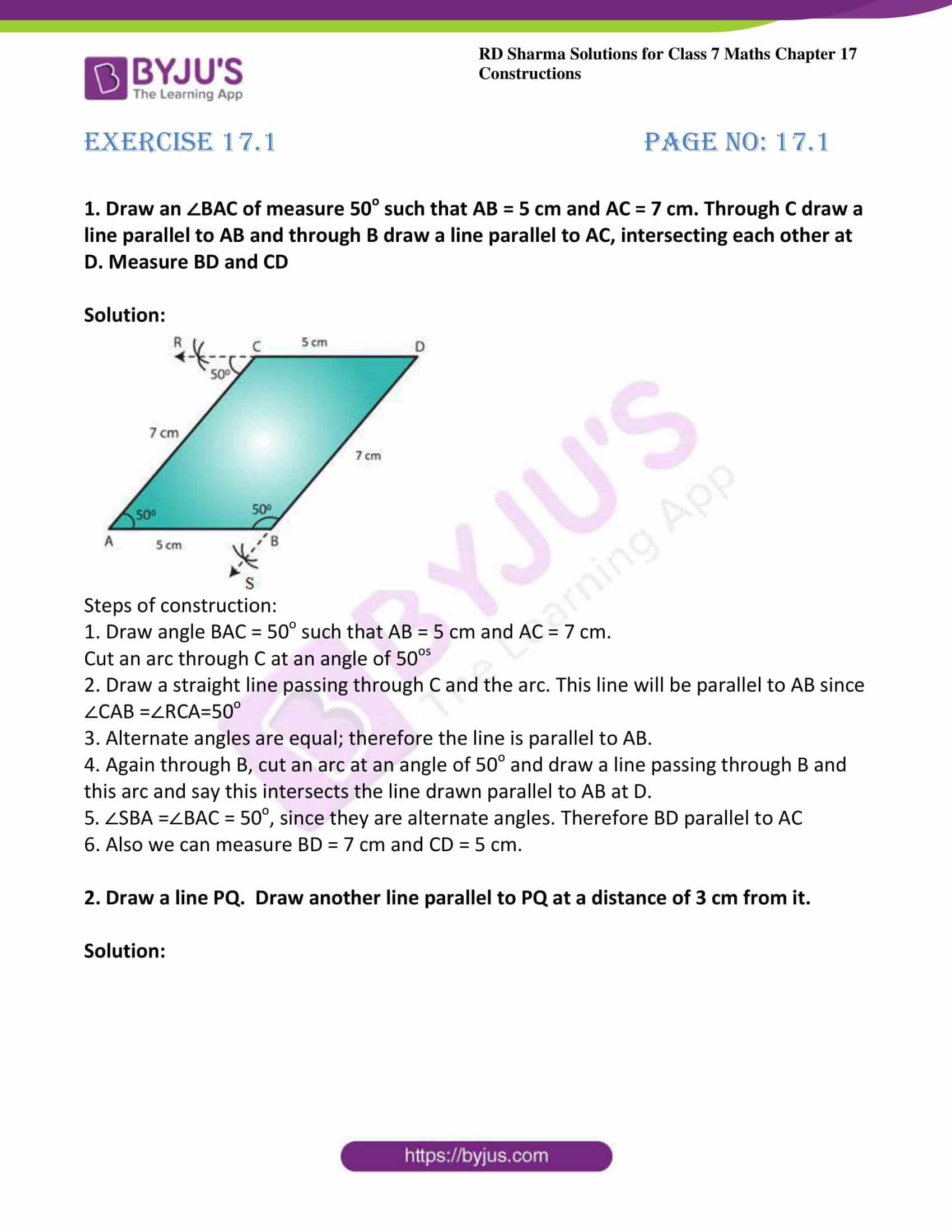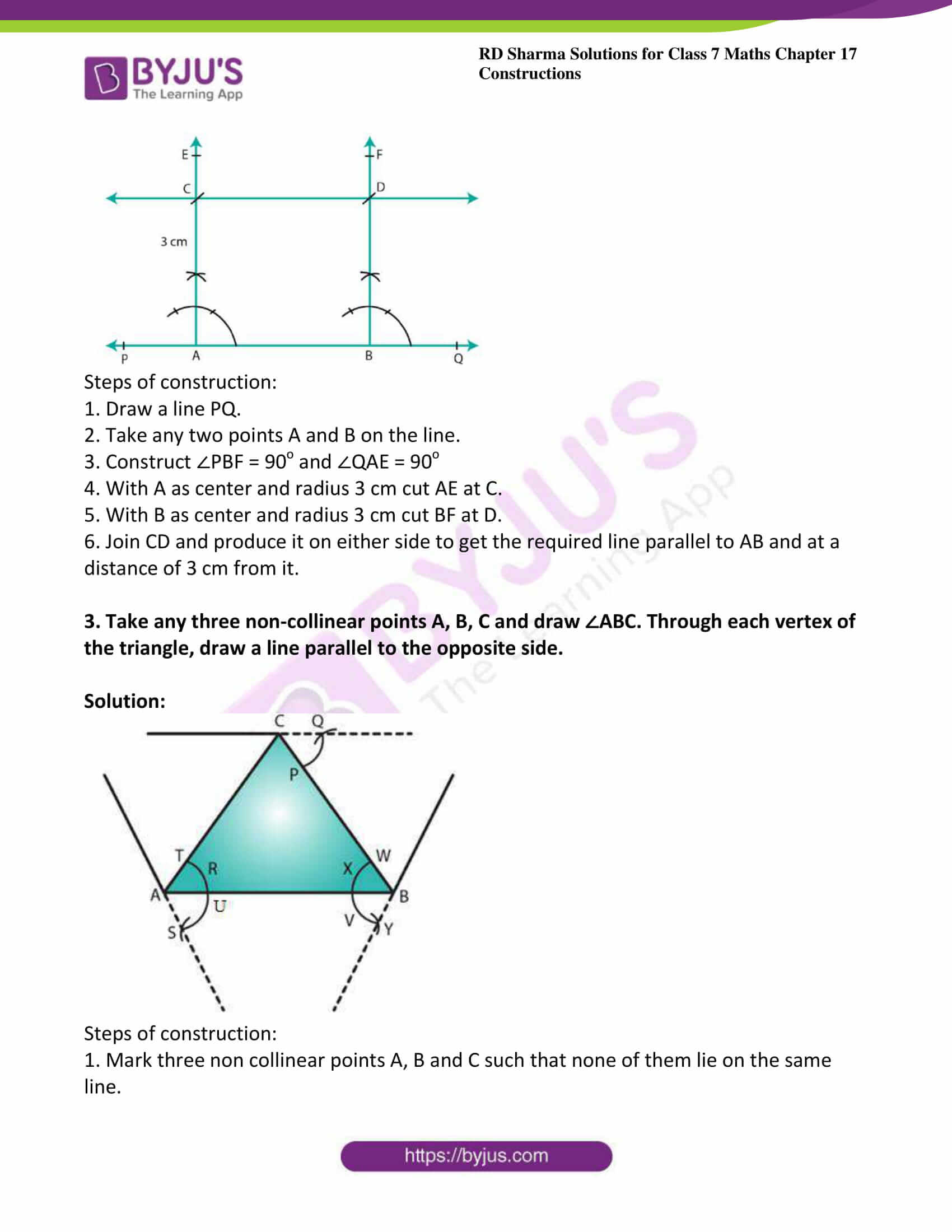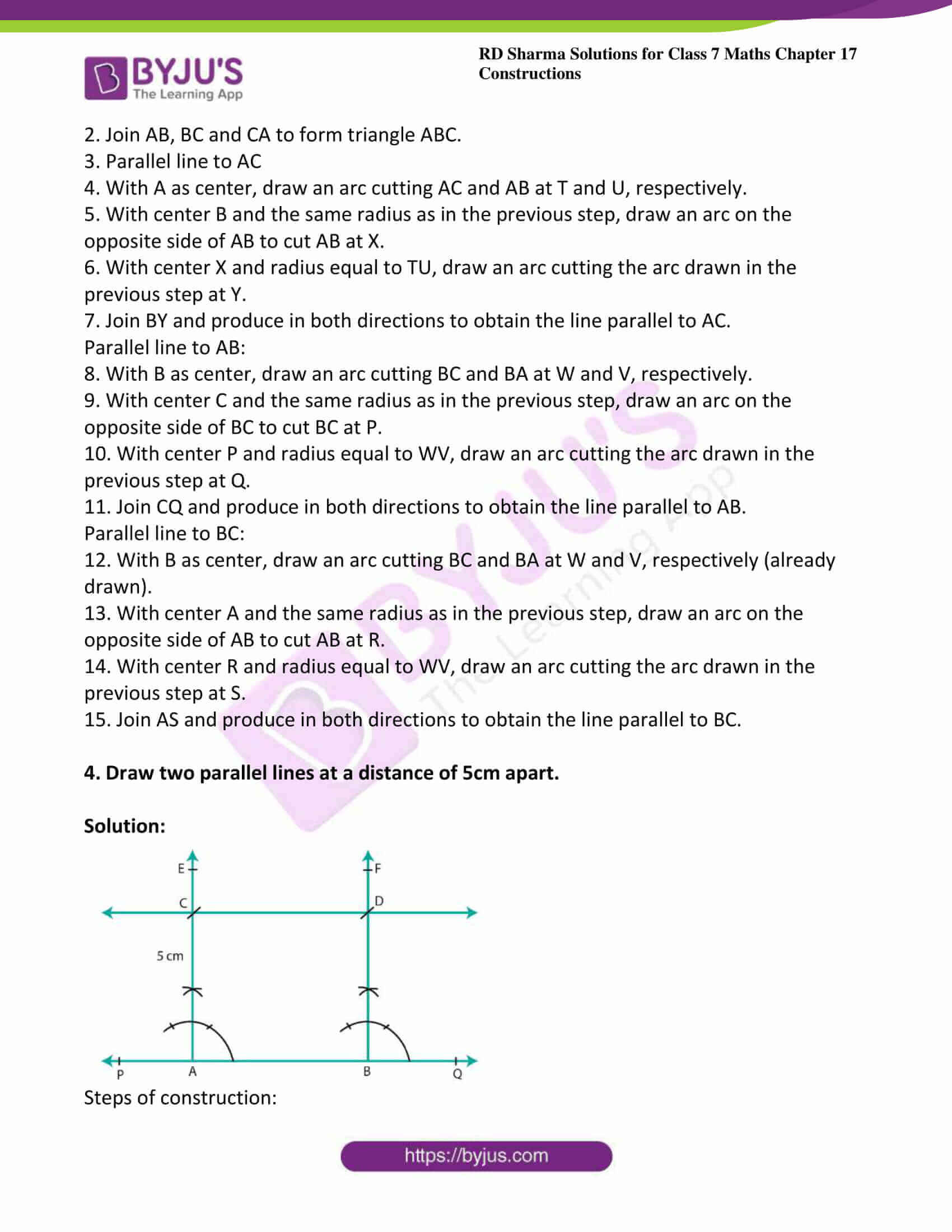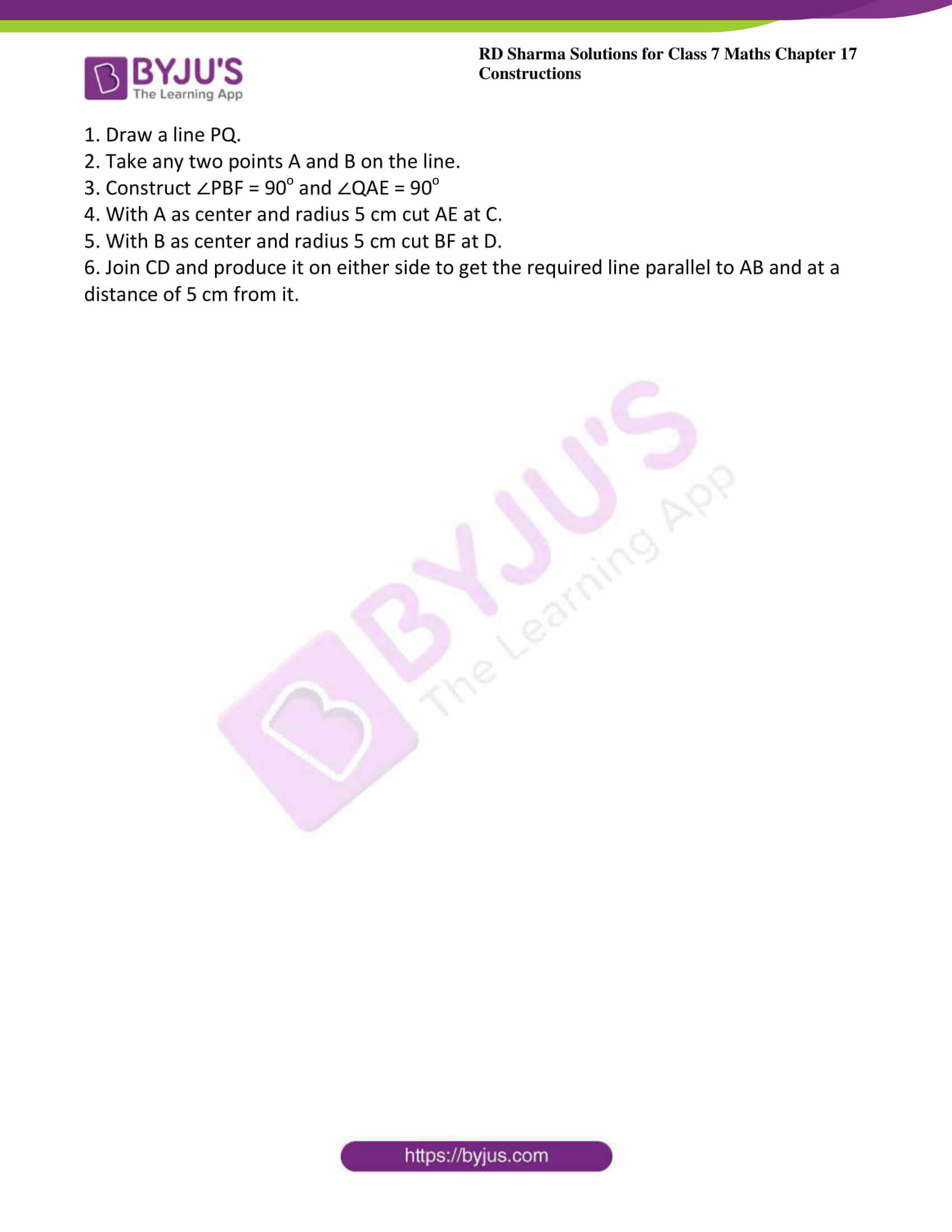### Access answers to Maths RD Sharma Solutions For Class 7 Chapter 17 – Constructions Exercise 17.1

Exercise 17.1 Page No: 17.1

1. Draw an ∠BAC of measure 50o such that AB = 5 cm and AC = 7 cm. Through C draw a line parallel to AB and through B draw a line parallel to AC, intersecting each other at D. Measure BD and CD

Solution: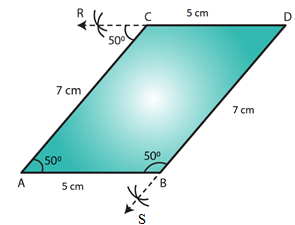Steps of construction:

1. Draw angle BAC = 50o such that AB = 5 cm and AC = 7 cm.

Cut an arc through C at an angle of 50os

2. Draw a straight line passing through C and the arc. This line will be parallel to AB since ∠CAB =∠RCA=50o

3. Alternate angles are equal; therefore the line is parallel to AB.

4. Again through B, cut an arc at an angle of 50o and draw a line passing through B and this arc and say this intersects the line drawn parallel to AB at D.

5. ∠SBA =∠BAC = 50o, since they are alternate angles. Therefore BD parallel to AC

6. Also we can measure BD = 7 cm and CD = 5 cm.

2. Draw a line PQ.  Draw another line parallel to PQ at a distance of 3 cm from it.

Solution: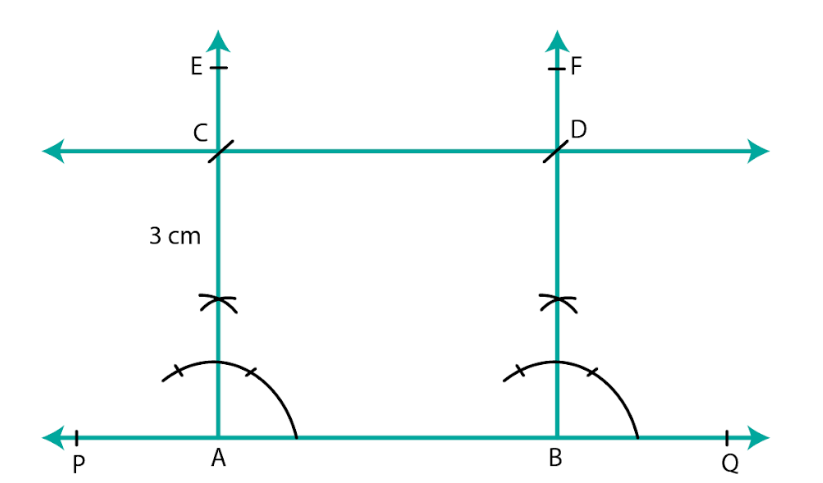Steps of construction:

1. Draw a line PQ.

2. Take any two points A and B on the line.

3. Construct ∠PBF = 90o and ∠QAE = 90o

4. With A as center and radius 3 cm cut AE at C.

5. With B as center and radius 3 cm cut BF at D.

6. Join CD and produce it on either side to get the required line parallel to AB and at a distance of 3 cm from it.

3. Take any three non-collinear points A, B, C and draw ∠ABC. Through each vertex of the triangle, draw a line parallel to the opposite side.

Solution: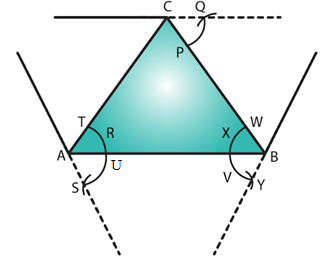Steps of construction:

1. Mark three non collinear points A, B and C such that none of them lie on the same line.

2. Join AB, BC and CA to form triangle ABC.

3. Parallel line to AC

4. With A as center, draw an arc cutting AC and AB at T and U, respectively.

5. With center B and the same radius as in the previous step, draw an arc on the opposite side of AB to cut AB at X.

6. With center X and radius equal to TU, draw an arc cutting the arc drawn in the previous step at Y.

7. Join BY and produce in both directions to obtain the line parallel to AC.

Parallel line to AB:

8. With B as center, draw an arc cutting BC and BA at W and V, respectively.

9. With center C and the same radius as in the previous step, draw an arc on the opposite side of BC to cut BC at P.

10. With center P and radius equal to WV, draw an arc cutting the arc drawn in the previous step at Q.

11. Join CQ and produce in both directions to obtain the line parallel to AB.

Parallel line to BC:

12. With B as center, draw an arc cutting BC and BA at W and V, respectively (already drawn).

13. With center A and the same radius as in the previous step, draw an arc on the opposite side of AB to cut AB at R.

14. With center R and radius equal to WV, draw an arc cutting the arc drawn in the previous step at S.

15. Join AS and produce in both directions to obtain the line parallel to BC.

4. Draw two parallel lines at a distance of 5cm apart.

Solution: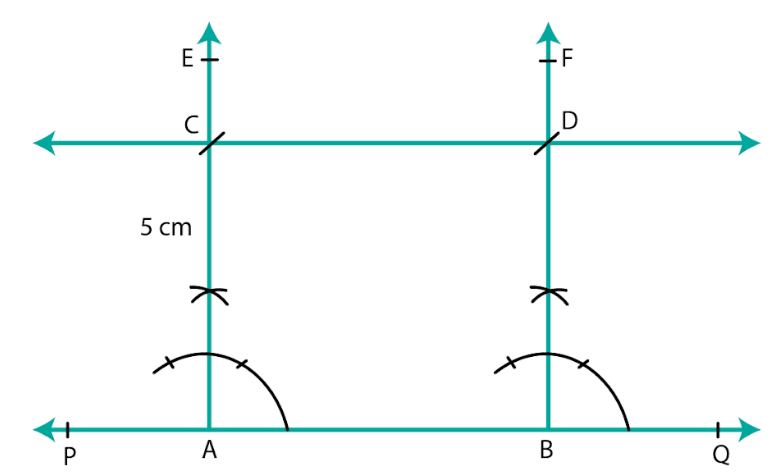Steps of construction:

1. Draw a line PQ.

2. Take any two points A and B on the line.

3. Construct ∠PBF = 90o and ∠QAE = 90o

4. With A as center and radius 5 cm cut AE at C.

5. With B as center and radius 5 cm cut BF at D.

6. Join CD and produce it on either side to get the required line parallel to AB and at a distance of 5 cm from it.